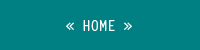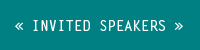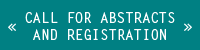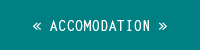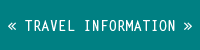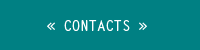Subject   We propose a six days long conference on Advance in Mathematical Fluid Mechanics to be held at Lisbon, Instituto para a Investigação Interdisciplinar da Universidade de  Lisboa, from June 30 to July 05 of 2014 having the Mathematical Physics Group (GFMUL) as its host. This scientific meeting will be a Satellite Conference of the 10th AIMS Conference on Dynamical Systems Differential Equations and Applications, July 07-July 11, 2014, Madrid, Spain. The aim of this scientific meeting is to present and discuss recent progresses in the Stochastic & Deterministic theory of Navier-Stokes equations, Euler equations and related problems. Among the questions addressed in the discussions are recent mathematical models in hydrodynamics, statistical description of the evolution, optimal transportation, deterministic and stochastic control problems in fluid dynamics, Vlasov equation and hydrodynamic limits, turbulent behaviour of flows, existence and regularity of solutions. Contributions from the two different branches of mathematics: PDE’s and Stochastic Analysis should give new insight on the understanding of the complex and uncertain nature of Fluid Dynamics. We hope that this meeting will facilitate the exchange of ideas between experts in Mathematical Fluid Dynamics and Stochastic Evolution Equations. The conference will include the following topics: Thermodynamics and Statistical Mechanics. The physical foundations and heuristics for stochastic and deterministic approaches in fluid mechanics. Vlasov equations and hydrodynamic limits. Variational methods in Fluids (deterministic and stochastic). Optimal Transport in fluid mechanics. Well-posedness of solutions. Boundary conditions: no-slip, slip, artificial. Boundary layers. Navier-Stokes's, Euler's, Prandtl's and related equations. Zero viscosity limit and large deviation techniques.